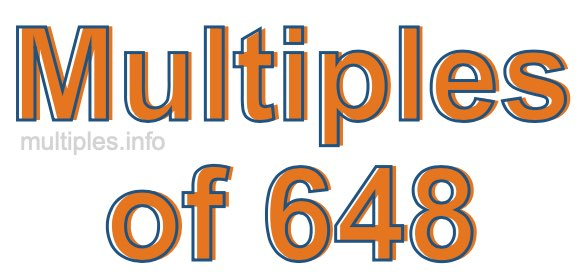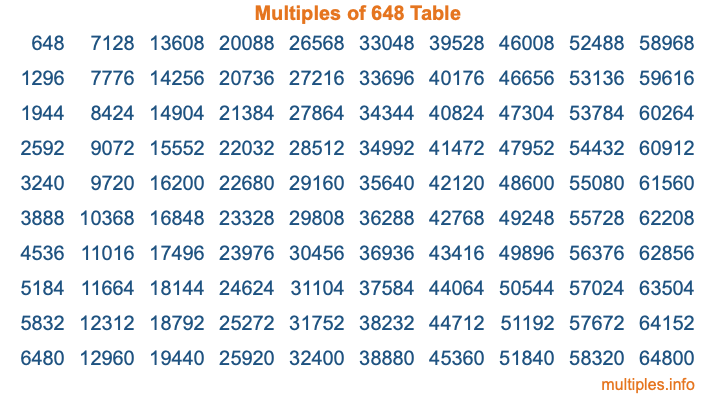Multiples of 648Welcome to the Multiples of 648 page. Here we will first teach you everything you will ever need to know about the multiples of 648, and then give you a study guide summary of everything we taught you to make sure you remember it all. Use this page to look up facts and learn information about the multiples of 648. This page will make you a multiples of six hundred forty-eight expert!

Definition of Multiples of 648
Multiples of 648 are all the numbers that when divided by 648 equal an integer. Each of the multiples of 648 are called a multiple. A multiple of 648 is created by multiplying 648 by an integer.

Therefore, to create a list of multiples of 648, you start with 1 multiplied by 648, then 2 multiplied by 648, then 3 multiplied by 648, and so on for as long as you want. Thus, the list of the first five multiples of 648 is 648, 1296, 1944, 2592, and 3240. To see a larger list of multiples of 648, see the printable image of Multiples of 648 further down on this page. We also have a category where you can choose any nth multiple of 648.

Multiples of 648 Checker
The Multiples of 648 Checker below checks to see if any number of your choice is a multiple of 648. In other words, it checks to see if there is any number (integer) that when multiplied by 648 will equal your number. To do that, we divide your number by 648. If the the quotient is an integer, then your number is a multiple of 648.

Is  a multiple of 648?

Least Common Multiple of 648 and ...
A Least Common Multiple (LCM) is the lowest multiple that two or more numbers have in common. This is also called the smallest common multiple or lowest common multiple and is useful to know when you are adding our subtracting fractions. Enter one or more numbers below (648 is already entered) to find the LCM.

Check out our LCM Calculator if you need more details about the Least Common Multiple or if you need the LCM for different numbers for adding and subtraction fractions.

nth Multiple of 648
As we stated above, 648 is the first multiple of 648, 1296 is the second multiple of 648, 1944 is the third multiple of 648, and so on. Enter a number below to find the nth multiple of 648.

th multiple of 648

Multiples of 648 vs Factors of 648
648 is a multiple of 648 and a factor of 648, but that is where the similarities end. All postive multiples of 648 are 648 or greater than 648. All positive factors of 648 are 648 or less than 648.

Below is the beginning list of multiples of 648 and the factors of 648 so you can compare:

Multiples of 648: 648, 1296, 1944, 2592, 3240, etc.

Factors of 648: 1, 2, 3, 4, 6, 8, 9, 12, 18, 24, 27, 36, 54, 72, 81, 108, 162, 216, 324, 648

As you can see, the multiples of 648 are all the numbers that you can divide by 648 to get a whole number. The factors of 648, on the other hand, are all the whole numbers that you can multiply by another whole number to get 648.

It's also interesting to note that if a number (x) is a factor of 648, then 648 will also be a multiple of that number (x).

Multiples of 648 vs Divisors of 648
The divisors of 648 are all the integers that 648 can be divided by evenly. Below is a list of the divisors of 648.

Divisors of 648: 1, 2, 3, 4, 6, 8, 9, 12, 18, 24, 27, 36, 54, 72, 81, 108, 162, 216, 324, 648

The interesting thing to note here is that if you take any multiple of 648 and divide it by a divisor of 648, you will see that the quotient is an integer.

Multiples of 648 Table
Below is an image of the first 100 multiples of 648 in a table. The table is in chronological order, column by column. The first column has the first ten multiples of 648, the second column has the next ten multiples of 648, and so on.The Multiples of 648 Table is also referred to as the 648 Times Table or Times Table of 648. You are welcome to print out our table for your studies.

Negative Multiples of 648
Although not often discussed or needed in math, it is worth mentioning that you can make a list of negative multiples of 648 by multiplying 648 by -1, then by -2, then by -3, and so on, to get the following list of negative multiples of 648:

-648, -1296, -1944, -2592, -3240, etc.

Multiples of 648 Summary
Below is a summary of important Multiples of 648 facts that we have discussed on this page. To retain the knowledge on this page, we recommend that you read through the summary and explain to yourself or a study partner why they hold true.

There are an infinite number of multiples of 648.

A multiple of 648 divided by 648 will equal a whole number.

648 divided by a factor of 648 equals a divisor of 648.

The nth multiple of 648 is n times 648.

The largest factor of 648 is equal to the first positive multiple of 648.

648 is a multiple of every factor of 648.

648 is a multiple of 648.

A multiple of 648 divided by a divisor of 648 equals an integer.

648 divided by a divisor of 648 equals a factor of 648.

Any integer times 648 will equal a multiple of 648.

Multiples of a Number
Here you can get the multiples of another number, all with the same attention to detail as we did for multiples of 648 on this page.

Multiples of
Multiples of 649
Did you find our page about multiples of six hundred forty-eight educational? Do you want more knowledge? Check out the multiples of the next number on our list!